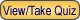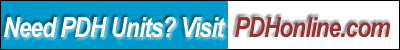Print this page

Course Outline

1. Learning Objective
2. Course Introduction
3. Course Content
4. Course Summary

This course includes a multiple choice quiz at the end.

Learning Objective

At the conclusion of this course, the student will:

• Be familiar with the interface, graphics, text and computational features of Mathcad
• Be able to perform engineering calculations using Mathcad

Course Introduction

Math is everywhere and in everything. From bridges to high-rises, from roller-coasters to the space shuttle, math is critical to today's high-tech society. Mathcad by PTC makes math easy and approachable for users from high school students to engineers who are at the cutting edge of some of the most important and demanding technical environments in the world.

Course Content

Mathcad is a valuable technical computing tool for engineers and architects. You can think of Mathcad as a combination of

•  a powerful technical computing environment centered on real math notation, and
•  a flexible, full-featured technical word processor.

Mathcad provides a rich problem-solving environment that gives you a wide variety of analysis and visualization tools. Unlike other technical software, Mathcad does math the same way you do. That is because it looks and works like a scratchpad and pencil. Mathcad's on-screen interface is a blank worksheet on which you can enter equations, graph data or functions, and annotate with text - anywhere on the worksheet.

Some of the important computational features of Mathcad for engineering applications are:

•  Built-in units of measurement and dimension checking.
•  Built-in solver for simultaneous equations and inequalities.
•  Summations, products and iteration.
•  Trigonometric, hyperbolic, exponential and Bessel functions.
•  Fast Fourier transformation, both one and two dimensional.
•  User-defined functions.
•  Vectors and matrices, including operation for matrix multiplication, matrix inverse, tranpose, determinant, dot and cross product.
Mathematical expressions in Mathcad look the way you would see them in a textbook or a reference book. The only difference is that Mathcad's equations and graphs are live, which means Mathcad recalculates the math and redraws the graphs every time you change any data, variable, or equation in a worksheet. This feature in Mathcad is called "Live Document Interface".

In addition to the Live Document Interface, another important feature that sets Mathcad apart from other technical computing tools is its built-in support for units. Mathcad can handle unit conversion automatically within a worksheet.

Therefore, the technical documents prepared using Mathcad can be easily understood and verified by others. In addition, using Mathcad in your work can relieve you from tedious and repetitive design calculations, eliminate arithmetic errors, and produce quality design calculations.

In this lesson, you will learn the basic features of Mathcad and two Mathcad applications in the real-world of the engineering analysis.

To learn the basic features of Mathcad, you need to purchase a copy of Mathcad from Mathcad.com. Once you install the software, you need to study the Mathcad's built-in tutorial (a PDF file, 688 KB, 51 pages). In addition, you need to watch the following video lessons:

(12:42 video)
2. Mathcad Prime 1.0 Demo (11:26 video)
3. Mathcad Prime 2.0 Webinar (1:00:15 video)
4. Concrete Beam Analysis using a Mathcad sheet (15:17 video)

In particular, you need to learn how to:

1. enter text
2. build mathematical expressions
3. define variables
4. define functions
5. define range variables
6. create graphs
7. specify units

Mathcad Web Library contains many examples of Mathcad applications in the various disciplines. In this lesson, you need to download and study the following two Mathcad application files since you will be asked to solve some simple beam problems when taking the quiz.

1. Elastic bending of beams with a rectangular cross section (bending.mcd -free download, Mathcad 2000i, 24 kb)

2. Shear and moment diagrams for any simply supported/overhanging beams (ssbeam.mcd-free download, Mathcad 7 Prof., 508 kb) by K. Hauck
Mathcad's flexible calculation and documentation environment is evolving as one of the most popular platforms for industry-specific solutions, which includes Mathcad Electronic Books, Mathcad Extension Packs, Dynamically Linked Libraries (DLL, i.e., user-created C++ libraries) and Interoperability with other application such as spreadsheet software.

Course Summary

This course covers some of the basic features of Mathcad, which include interface features, graphical features, computational features, and text and document features. Through this online course, the student should be able to use Mathcad to solve some of the routine design problems in his/her design practice. For more advanced topics on Mathcad applications such as electronic books and animation, you may visit the Mathsoft.com website.